# image compression Tech. 31.pptx

2 Jun 2023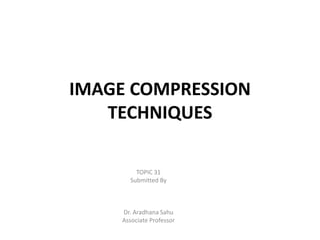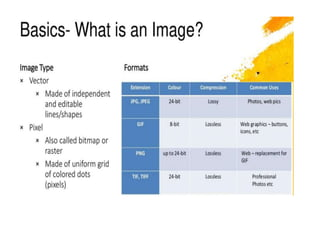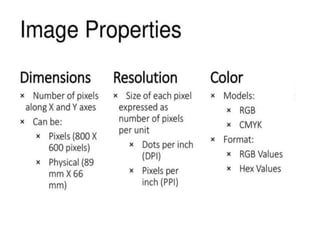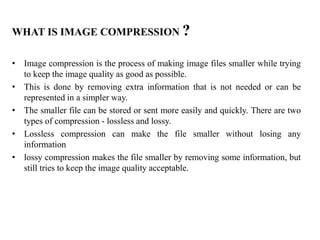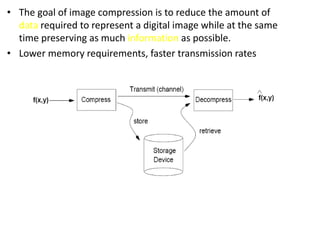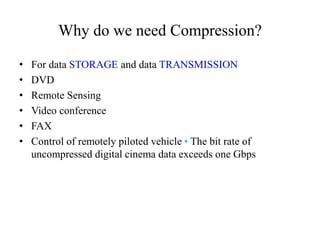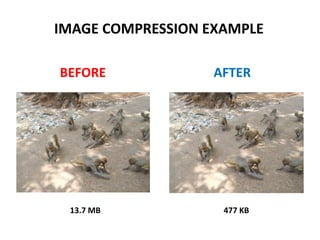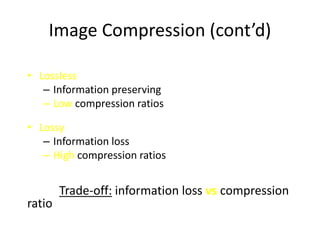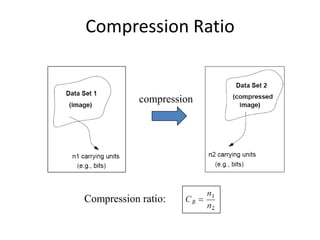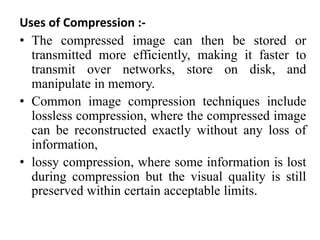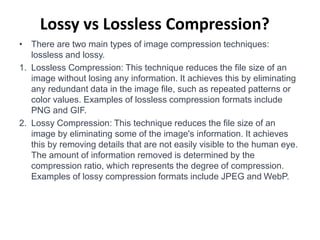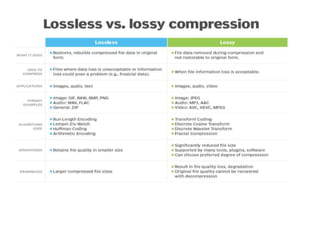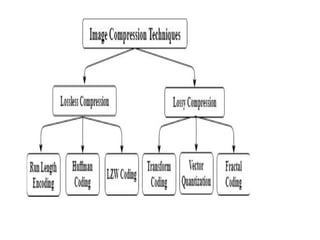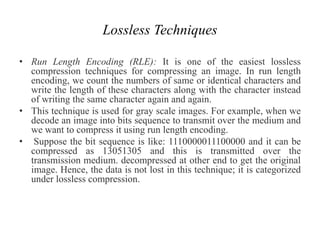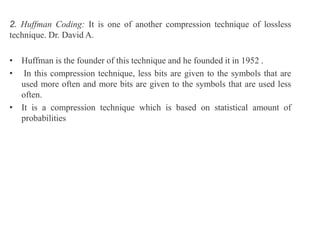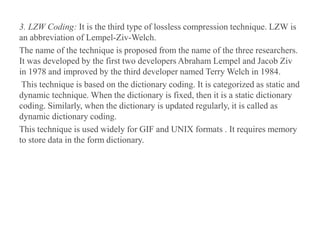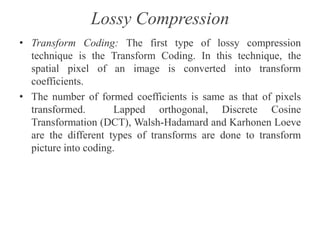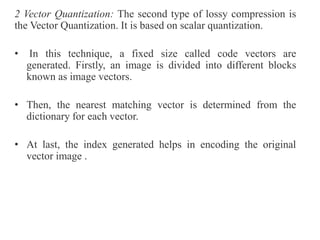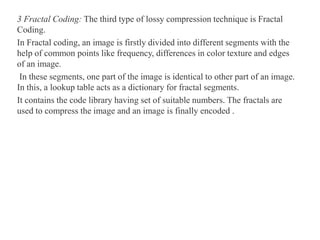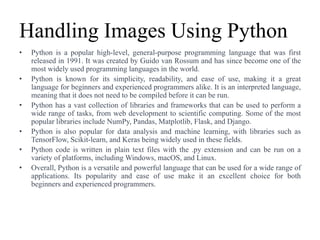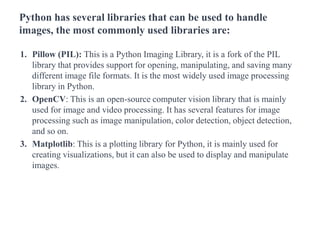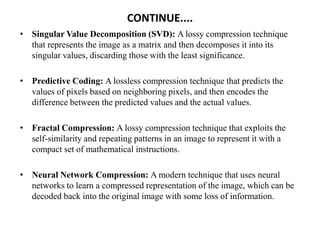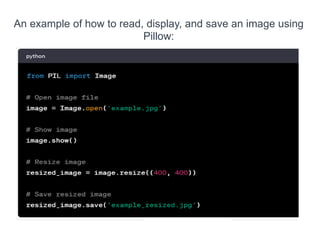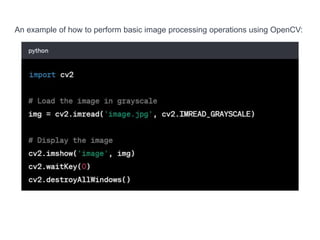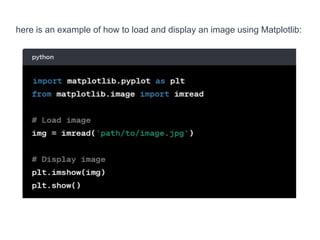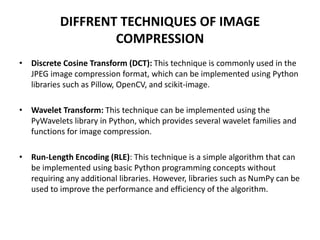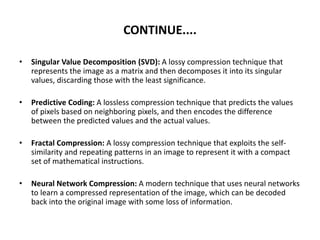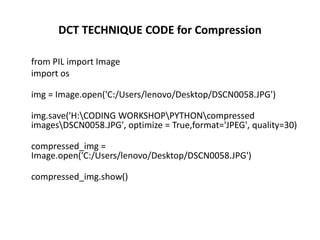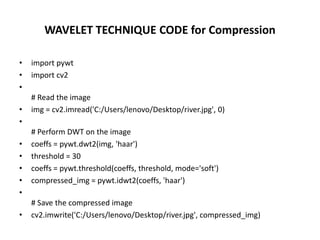1 sur 29

### image compression Tech. 31.pptx

• 1. IMAGE COMPRESSION TECHNIQUES TOPIC 31 Submitted By Dr. Aradhana Sahu Associate Professor
• 4. WHAT IS IMAGE COMPRESSION ? • Image compression is the process of making image files smaller while trying to keep the image quality as good as possible. • This is done by removing extra information that is not needed or can be represented in a simpler way. • The smaller file can be stored or sent more easily and quickly. There are two types of compression - lossless and lossy. • Lossless compression can make the file smaller without losing any information • lossy compression makes the file smaller by removing some information, but still tries to keep the image quality acceptable.
• 5. • The goal of image compression is to reduce the amount of data required to represent a digital image while at the same time preserving as much information as possible. • Lower memory requirements, faster transmission rates
• 6. Why do we need Compression? • For data STORAGE and data TRANSMISSION • DVD • Remote Sensing • Video conference • FAX • Control of remotely piloted vehicle • The bit rate of uncompressed digital cinema data exceeds one Gbps
• 7. IMAGE COMPRESSION EXAMPLE BEFORE AFTER 13.7 MB 477 KB
• 8. Image Compression (cont’d) • Lossless – Information preserving – Low compression ratios • Lossy – Information loss – High compression ratios Trade-off: information loss vs compression ratio
• 10. Uses of Compression :- • The compressed image can then be stored or transmitted more efficiently, making it faster to transmit over networks, store on disk, and manipulate in memory. • Common image compression techniques include lossless compression, where the compressed image can be reconstructed exactly without any loss of information, • lossy compression, where some information is lost during compression but the visual quality is still preserved within certain acceptable limits.
• 11. Lossy vs Lossless Compression? • There are two main types of image compression techniques: lossless and lossy. 1. Lossless Compression: This technique reduces the file size of an image without losing any information. It achieves this by eliminating any redundant data in the image file, such as repeated patterns or color values. Examples of lossless compression formats include PNG and GIF. 2. Lossy Compression: This technique reduces the file size of an image by eliminating some of the image's information. It achieves this by removing details that are not easily visible to the human eye. The amount of information removed is determined by the compression ratio, which represents the degree of compression. Examples of lossy compression formats include JPEG and WebP.
• 14. Lossless Techniques • Run Length Encoding (RLE): It is one of the easiest lossless compression techniques for compressing an image. In run length encoding, we count the numbers of same or identical characters and write the length of these characters along with the character instead of writing the same character again and again. • This technique is used for gray scale images. For example, when we decode an image into bits sequence to transmit over the medium and we want to compress it using run length encoding. • Suppose the bit sequence is like: 1110000011100000 and it can be compressed as 13051305 and this is transmitted over the transmission medium. decompressed at other end to get the original image. Hence, the data is not lost in this technique; it is categorized under lossless compression.
• 15. 2. Huffman Coding: It is one of another compression technique of lossless technique. Dr. David A. • Huffman is the founder of this technique and he founded it in 1952 . • In this compression technique, less bits are given to the symbols that are used more often and more bits are given to the symbols that are used less often. • It is a compression technique which is based on statistical amount of probabilities
• 16. 3. LZW Coding: It is the third type of lossless compression technique. LZW is an abbreviation of Lempel-Ziv-Welch. The name of the technique is proposed from the name of the three researchers. It was developed by the first two developers Abraham Lempel and Jacob Ziv in 1978 and improved by the third developer named Terry Welch in 1984. This technique is based on the dictionary coding. It is categorized as static and dynamic technique. When the dictionary is fixed, then it is a static dictionary coding. Similarly, when the dictionary is updated regularly, it is called as dynamic dictionary coding. This technique is used widely for GIF and UNIX formats . It requires memory to store data in the form dictionary.
• 17. Lossy Compression • Transform Coding: The first type of lossy compression technique is the Transform Coding. In this technique, the spatial pixel of an image is converted into transform coefficients. • The number of formed coefficients is same as that of pixels transformed. Lapped orthogonal, Discrete Cosine Transformation (DCT), Walsh-Hadamard and Karhonen Loeve are the different types of transforms are done to transform picture into coding.
• 18. 2 Vector Quantization: The second type of lossy compression is the Vector Quantization. It is based on scalar quantization. • In this technique, a fixed size called code vectors are generated. Firstly, an image is divided into different blocks known as image vectors. • Then, the nearest matching vector is determined from the dictionary for each vector. • At last, the index generated helps in encoding the original vector image .
• 19. 3 Fractal Coding: The third type of lossy compression technique is Fractal Coding. In Fractal coding, an image is firstly divided into different segments with the help of common points like frequency, differences in color texture and edges of an image. In these segments, one part of the image is identical to other part of an image. In this, a lookup table acts as a dictionary for fractal segments. It contains the code library having set of suitable numbers. The fractals are used to compress the image and an image is finally encoded .
• 20. Handling Images Using Python • Python is a popular high-level, general-purpose programming language that was first released in 1991. It was created by Guido van Rossum and has since become one of the most widely used programming languages in the world. • Python is known for its simplicity, readability, and ease of use, making it a great language for beginners and experienced programmers alike. It is an interpreted language, meaning that it does not need to be compiled before it can be run. • Python has a vast collection of libraries and frameworks that can be used to perform a wide range of tasks, from web development to scientific computing. Some of the most popular libraries include NumPy, Pandas, Matplotlib, Flask, and Django. • Python is also popular for data analysis and machine learning, with libraries such as TensorFlow, Scikit-learn, and Keras being widely used in these fields. • Python code is written in plain text files with the .py extension and can be run on a variety of platforms, including Windows, macOS, and Linux. • Overall, Python is a versatile and powerful language that can be used for a wide range of applications. Its popularity and ease of use make it an excellent choice for both beginners and experienced programmers.
• 21. Python has several libraries that can be used to handle images, the most commonly used libraries are: 1. Pillow (PIL): This is a Python Imaging Library, it is a fork of the PIL library that provides support for opening, manipulating, and saving many different image file formats. It is the most widely used image processing library in Python. 2. OpenCV: This is an open-source computer vision library that is mainly used for image and video processing. It has several features for image processing such as image manipulation, color detection, object detection, and so on. 3. Matplotlib: This is a plotting library for Python, it is mainly used for creating visualizations, but it can also be used to display and manipulate images.
• 22. CONTINUE.... • Singular Value Decomposition (SVD): A lossy compression technique that represents the image as a matrix and then decomposes it into its singular values, discarding those with the least significance. • Predictive Coding: A lossless compression technique that predicts the values of pixels based on neighboring pixels, and then encodes the difference between the predicted values and the actual values. • Fractal Compression: A lossy compression technique that exploits the self-similarity and repeating patterns in an image to represent it with a compact set of mathematical instructions. • Neural Network Compression: A modern technique that uses neural networks to learn a compressed representation of the image, which can be decoded back into the original image with some loss of information.
• 23. An example of how to read, display, and save an image using Pillow:
• 24. An example of how to perform basic image processing operations using OpenCV:
• 25. here is an example of how to load and display an image using Matplotlib:
• 26. DIFFRENT TECHNIQUES OF IMAGE COMPRESSION • Discrete Cosine Transform (DCT): This technique is commonly used in the JPEG image compression format, which can be implemented using Python libraries such as Pillow, OpenCV, and scikit-image. • Wavelet Transform: This technique can be implemented using the PyWavelets library in Python, which provides several wavelet families and functions for image compression. • Run-Length Encoding (RLE): This technique is a simple algorithm that can be implemented using basic Python programming concepts without requiring any additional libraries. However, libraries such as NumPy can be used to improve the performance and efficiency of the algorithm.
• 27. CONTINUE.... • Singular Value Decomposition (SVD): A lossy compression technique that represents the image as a matrix and then decomposes it into its singular values, discarding those with the least significance. • Predictive Coding: A lossless compression technique that predicts the values of pixels based on neighboring pixels, and then encodes the difference between the predicted values and the actual values. • Fractal Compression: A lossy compression technique that exploits the self- similarity and repeating patterns in an image to represent it with a compact set of mathematical instructions. • Neural Network Compression: A modern technique that uses neural networks to learn a compressed representation of the image, which can be decoded back into the original image with some loss of information.
• 28. DCT TECHNIQUE CODE for Compression from PIL import Image import os img = Image.open('C:/Users/lenovo/Desktop/DSCN0058.JPG') img.save('H:CODING WORKSHOPPYTHONcompressed imagesDSCN0058.JPG', optimize = True,format='JPEG', quality=30) compressed_img = Image.open('C:/Users/lenovo/Desktop/DSCN0058.JPG') compressed_img.show()
• 29. WAVELET TECHNIQUE CODE for Compression • import pywt • import cv2 • # Read the image • img = cv2.imread('C:/Users/lenovo/Desktop/river.jpg', 0) • # Perform DWT on the image • coeffs = pywt.dwt2(img, 'haar') • threshold = 30 • coeffs = pywt.threshold(coeffs, threshold, mode='soft') • compressed_img = pywt.idwt2(coeffs, 'haar') • # Save the compressed image • cv2.imwrite('C:/Users/lenovo/Desktop/river.jpg', compressed_img)